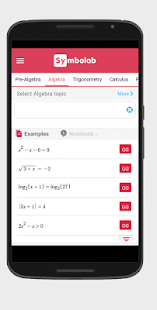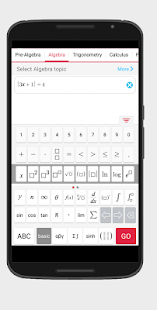# Symbolab – Math solver

#### 4.5.0Download APK
0/5 No votes
Developer
Symbolab
Version
4.5.0
Updated
November 20, 2018
Requirements
4.4 and up

## Description

Your private math tutor, solves any math problem with steps! equations, integrals, derivatives, , limits and much more.(Steps require a one-time in-app purchase)Symbolab Math Solver app is composed of over a hundred Symbolab most powerful calculators:Equation CalculatorIntegral CalculatorDerivative CalculatorLimit calculatorInequality CalculatorTrigonometry CalculatorMatrix CalculatorFunctions CalculatorSeries CalculatorODE CalculatorLaplace Transform CalculatorDownload the app to experience Symbolab full set of calculators.Symbolab Math Solver solves any math problem including Pre- Algebra, Algebra, Pre-Calculus, Calculus, Trigonometry, Functions, Matrix, Vectors, Geometry and Statistics.
* View graphs interactively in Symbolab Graphing Calculator* Enhancements and bug fixes

## What's new

* View graphs interactively in Symbolab Graphing Calculator* Enhancements and bug fixes

## Images